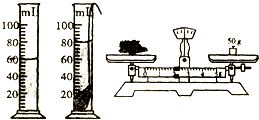# 利用浮力知识测量小石块的密度(学习了浮力知识后,小华找到了一种测小石块密度的新方法：先将小石)

## 1.学习了浮力知识后,小华找到了一种测小石块密度的新方法：先将小石

(1) ∵G=mg

∴m=G/g = 2N/10N/kg = 0.2kg （直接用弹簧测力计称出的就是石块的重力）

(2) F = G物 - G侵 = 2N - 1.5N =0.5N （浮力等于弹簧测力计在空气中的示数减去侵在水中时弹簧测力计的示数）

(3)F=p水gv排 v排=F/p水g =0.5N/(1*10^3 *10) = 0.5*10^-4 m^3

∵石块全部侵没在水中

∴V石 = v排=0.5*10^-4 m^3

(4) p=m/v=0.2kg /(0.5*10^-4 m^3) = 4*10^3 kg/m^3

## 2.小明和同学们在实验室中利用浮力的知识来测量物体的密度：（1）测

(1)由图可知，石块重为5N，石块浸没在水中弹簧测力计的示数为2.8N,

∵F浮=ρ水v排g，石块在水中下沉时，排开水的体积不变，

∴石块受到水的浮力不变，

∵G=F浮+F示，

∴F示=G-F浮，

∴弹簧测力计的示数不变.

(2)要测量物体的密度，一定想法测量物体的质量和体积.

F1

g .

F1+F2

ρ水g ,

m

V =

F1

g

F1+F2

ρ水g =

F1ρ水

F1+F2 .

(2)(a)向上拉动弹簧测力计，使物体完全浸没在水中静止时；（c）

F1ρ水

F1+F2 ;(d)增大吸盘的表面积.

## 3.测量小石块密度有多少种方法

1、利用浮力知识测密度：器材：弹簧测力计、细线、装水的烧杯。

B、用测力计测出石块全部进入水中时的“视重”G1;

2、利用密度定义公式：器材：天平、砝码；量筒、水；细线。（天平砝码亦可以换成弹簧秤）

B、用细线、量筒、水测出石块体积V。